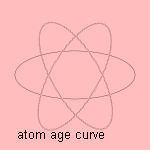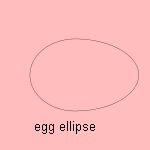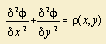# ellipse

## conic section

Make your own painting with this ellipse applet:The word ellipse is derived from the Greek word elleipsis (defect). The same defect do we find in the linguistic ellipse: omitting a part of a sentence, in situations that the part can be thought easily. So is the stimulation 'at work!' an linguistic ellipse.
Another fine quality of an ellipse is that for each point the sum of distances to the foci is constant. This gives us an easy way to construct (and to define) an ellipse
Fasten a rope in the two foci, and draw the curve with a pencil tight to the (strained) cord.
This quality leads to the bipolar equation given above.
In Cartesian coordinates the ellipse is to be written as: x˛ + a˛ y˛ = 1.An ellipse is a kind of oval, but a special one: it has two symmetry axes.
The curve is one of the conic section curves that results when slicing a conic.
An ellipse is also the result of the intersection of a (right circular) cylinder and a plane. As can be illustrated by the shape of the Tycho Brahe planetarium (situated in Kopenhagen, Denmark).

Menaichmos (350 BC) was the first to study the ellipse, Euclid wrote about the curve, and its name was given by Apollonius (200 BC).

The ellipse can be transformed into a circle by a linear transformation. It is the ellipse with the two axes equal in length. In fact, the ellipse can be seen as the form between the circle (eccentricity = 0) and the parabola (eccentricity = 1).

Some other qualities of the ellipse are the following:

Imagine an ellipse as a mirror strip. When you place a lamp at one focus, then there appears a light on the place of the other focus too. In Dutch the focus is designated with the word 'burning point', referring to this phenomenon. By the way, these foci always lay on the longer axis.

The astronomer Johann Kepler studied the celestial orbits. While he believed in 1602 the orbit of Mars was oval, later he found that the the form is elliptical, with the sun in the focus 1), what he published in 1609 in his work Astronomica Nova. His conclusion was based on the astronomical data of the astronomer Brahe. Theoretically it's the case of the two body problem 2). Newton was the first to derive the elliptical orbit out of the forces between planet and sun.
The planetary orbits are close to a circle, the eccentricity of the Mars orbit is about 1/11, the Earth orbit about 1/60.

Rotating the ellipse gives an ellipsoid, what is a good approximation of the shape of our planet earth.Comets can move in an elliptical orbit. Halley found in 1705 that the comet, which is now called after him, moved around the sun in an elliptical orbit. Its orbit is close to a parabola, having an eccentricity of about 0.9675.

On the much smaller scale of the atom one sees electrons orbiting round the atom kernels. A visual representation of this view is used to denote our atom age: the atom age curve.

The area of an ellipse has the value πab (where a and b are half of the axes).
The search for the arc length of an ellipse led to the so-called elliptic functions: the (incomplete) elliptic integral (of the second kind) gives an expression for this length.

The potential equation, a partial differential equation of second order, is also called the elliptical differential equation. This is because of the similarity between the equations of ellipse and differential equation 3). Normally mathematicians work in an Euclidean geometry, where the fifth Euclidean postulate is valid: given a line l and a point P, not on l; then there exists only one line parallel to l through P. When the assumption is that no such line exists, you enter a geometry that is called the elliptical geometry.
In multivariate analysis the Mahalanobis distance weights the differences in variability of the variables. Distances are measured in units of standard deviation. It is easy to see that the lines of constant standard deviation are ellipses.

Brocard pointed out the construction of a specific ellipse, inscribed in a triangle: Brocard's ellipse. The Steiner ellipse is the ellipse through the three vertices of a triangle and with the centroid of the triangle as its center.And in our universe we see that some (older) milky ways resemble an ellipse, they are called elliptical star constellations.
An egg can be formed by two halves of different ellipses: the egg ellipse.

notes

1) first Kepler's law.

2) Kuipers 1966 p. 370.

3) substitute the partial derivatives by x en y in: